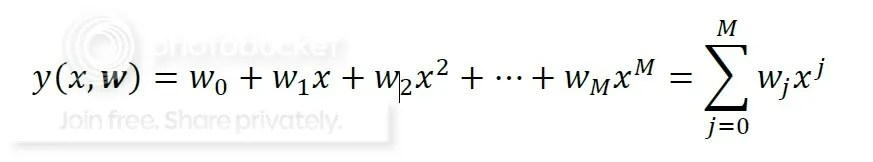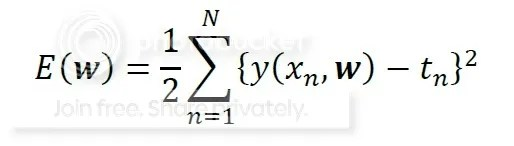# Polynomial Curve Fitting

Hi all, after sometimes far from update. I would like give some update today. :D Let’s we discuss about Linear regression. What is linear regression? I would try to explain using curve fitting problem.  Suppose we have 100 pair data, x and t. Where x is the input (1 dimension) and t is the target (1 dimension).

x: {?1,?2,…,?100} represents the input values and
t: {?1,?2,…,?100} represents the target values.

Let us spit them into training set and test set. Training set is the first 80 data and the test set is the last 20 data. We will fit the data by applying the following (model function) polynomial function.The goal is to identify the coefficients w (vector) such that y(x,w) ‘fits’ the data well. The ‘best’ curve has minimum error between curve and data points.  This is called the least squares approach, since we minimize the square of the error. The least squares  as followThis is the plot of original data.